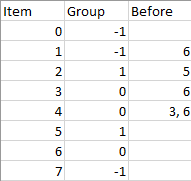# GeetCode Hub

There are `n` items each belonging to zero or one of `m` groups where `group[i]` is the group that the `i`-th item belongs to and it's equal to `-1` if the `i`-th item belongs to no group. The items and the groups are zero indexed. A group can have no item belonging to it.

Return a sorted list of the items such that:

• The items that belong to the same group are next to each other in the sorted list.
• There are some relations between these items where `beforeItems[i]` is a list containing all the items that should come before the `i`-th item in the sorted array (to the left of the `i`-th item).

Return any solution if there is more than one solution and return an empty list if there is no solution.

Example 1:```Input: n = 8, m = 2, group = [-1,-1,1,0,0,1,0,-1], beforeItems = [[],,,,[3,6],[],[],[]]
Output: [6,3,4,1,5,2,0,7]
```

Example 2:

```Input: n = 8, m = 2, group = [-1,-1,1,0,0,1,0,-1], beforeItems = [[],,,,,[],,[]]
Output: []
Explanation: This is the same as example 1 except that 4 needs to be before 6 in the sorted list.
```

Constraints:

• `1 <= m <= n <= 3 * 104`
• `group.length == beforeItems.length == n`
• `-1 <= group[i] <= m - 1`
• `0 <= beforeItems[i].length <= n - 1`
• `0 <= beforeItems[i][j] <= n - 1`
• `i != beforeItems[i][j]`
• `beforeItems[i] `does not contain duplicates elements.

class Solution { public int[] sortItems(int n, int m, int[] group, List<List<Integer>> beforeItems) { } }